# Interpretation of |z1-z2|

Interpretation of |z1-z2|

Let $${z_1}$$ and $${z_2}$$ represent two fixed points in the complex plane. There is a very useful way to interpret the expression $$\left| {{z_1} - {z_2}} \right|$$. Consider the following figure, which geometrically depicts the vector $${z_1} - {z_2}$$: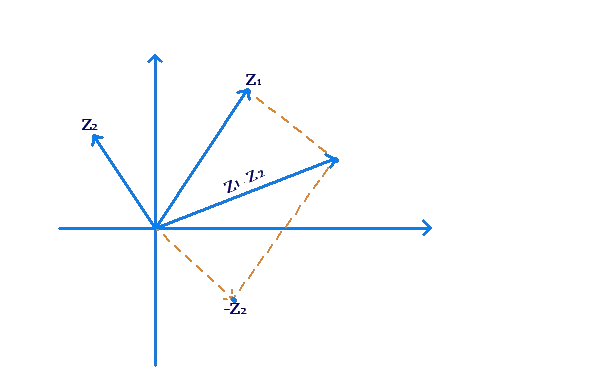However, observe that this vector is also equal to the vector drawn from the point $${z_2}$$ to the point $${z_1}$$: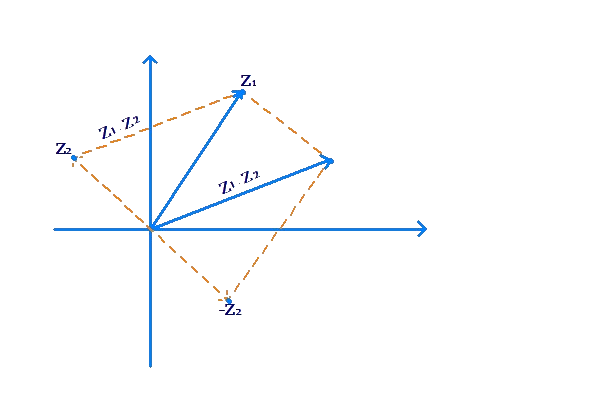Thus, $$\left| {{z_1} - {z_2}} \right|$$ represents the length of the vector drawn from $${z_2}$$ to $${z_1}$$. In other words, $$\left| {{z_1} - {z_2}} \right|$$ represents the distance between the points $${z_1}$$ and $${z_2}$$.

Let us take an example. Consider

\begin{align}&{z_1} = 1 + i\\&{z_2} = - 3i\end{align}

The expression $$\left| {{z_1} - {z_2}} \right|$$, as we concluded, represents the distance between the points $${z_1}$$ and $${z_2}$$, which is $$\sqrt {17}$$, as is evident from the following figure: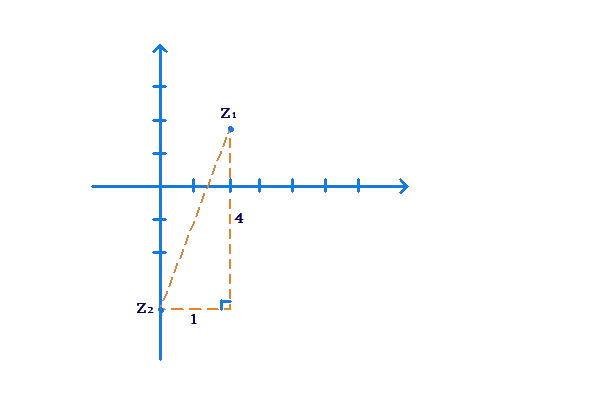We can verify this algebraically:

\begin{align}&{z_1} - {z_2} = \left( {1 + i} \right) - \left( { - 3i} \right) = 1 + 4i\\&\Rightarrow \,\,\,{z_1} - {z_2} = \sqrt {1 + 16} = \sqrt {17} \end{align}

This interpretation of the expression $$\left| {{z_1} - {z_2}} \right|$$ as the distance between the points $${z_1}$$ and $${z_2}$$ is extremely useful and powerful. Let us see how.

Suppose that z is a variable point in the complex plane such that $$\left| {z - i} \right| = 3$$. What is the locus of z? In other words, what path does z trace out, while satisfying this constraint?

We can interpret $$\left| {z - i} \right|$$ as the distance between the variable point z and the fixed point i. The equation $$\left| {z - i} \right| = 3$$ says that the variable point z moves in such a way so that it is always at a constant distance of 3 units from the fixed point i. Thus, z traces out a circle in the plane, with center as the point i and radius 3 units: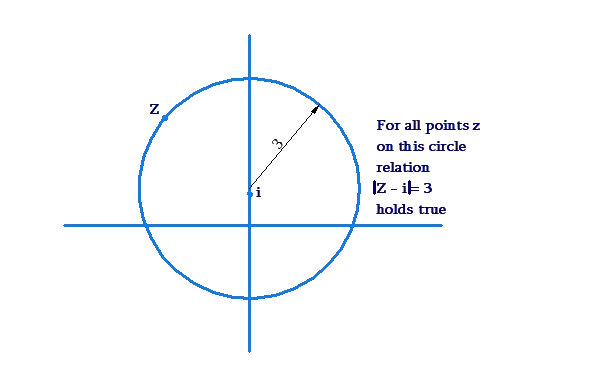Let’s take another example. Consider the equation

$\left| {z - 1 + i} \right| = 2$

We write this equation as

$\left| {z - \left( {1 - i} \right)} \right| = 2$

This says that the distance of z from the fixed point $$\left( {1 - i} \right)$$ is always 2 units. Thus, z traces out a circle in the plane, with center as the point $$\left( {1 - i} \right)$$ and radius equal to 2 units: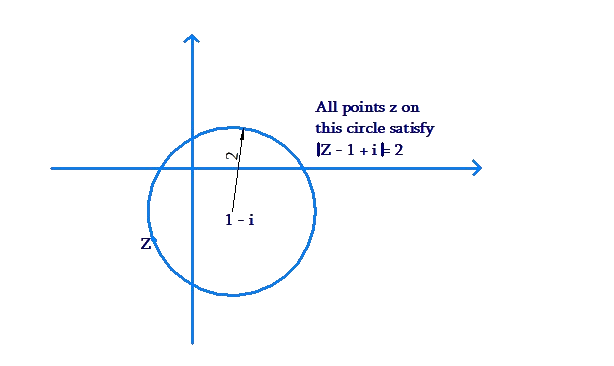Example 1: z is a variable point in the plane such that

$\left| {z - 2 + 3i} \right| = 11$

Plot the locus of z.

Solution: We rewrite the given equation as

$\left| {z - \left( {2 - 3i} \right)} \right| = 1$

Thus, z traces out a circle of radius 1 unit, centered at the point $$\left( {2 - 3i} \right)$$: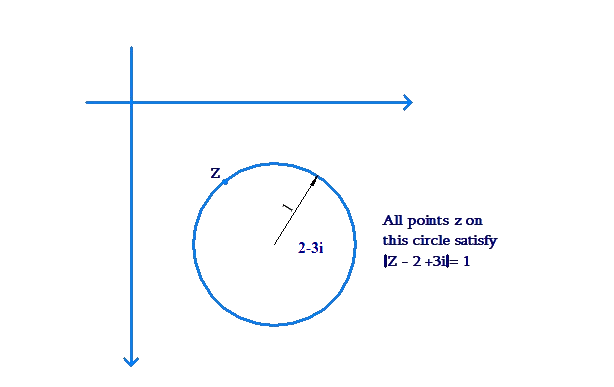Example 2: A variable point z always satisfies

$$\left| {z - i} \right| = \left| {z + i} \right|$$

As z moves, what path will it trace out in the plane?

Solution: First, we rewrite the given equation as

$\left| {z - i} \right| = \left| {z - \left( { - i} \right)} \right|$

This equation says that the distance of z from the point $$i$$ is equal to the distance of z from the point $$\left( { - i} \right)$$. Thus, z lies on the perpendicular bisector of these two points: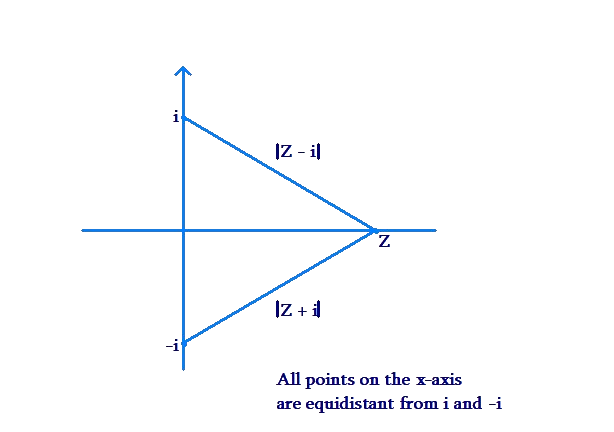Clealy, z can lie anywhere on the real axis.

Example 3: Plot the region in which z can lie, if it satisfied $$1 < \left| z \right| < 2$$.

Solution: We can interpret $$\left| z \right|$$ or $$\left| {z - 0} \right|$$ as the distance between the point z and the origin. The given inequality says that the distance of the point z from the origin is greater than 1 but less than 2. Thus, z can lie anywhere in the following ring-shaped region: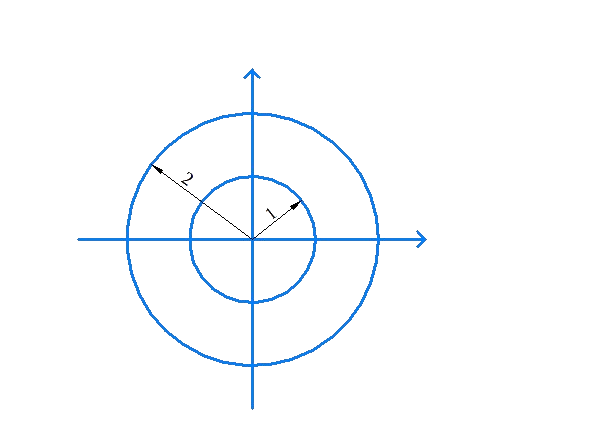Complex Numbers
grade 10 | Questions Set 1
Complex Numbers